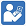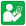Test Sheets - Multiply and Divide Fractions
Multiply and Divide Fractions - Test 1
Multiply and Divide Fractions - Test 2
Multiply and Divide Fractions - Test 3
Multiply and Divide Fractions - Test 4
Multiply and Divide Fractions - Test 5
Multiply and Divide Fractions - Test 6Practice Sheets - Multiply and Divide Fractions